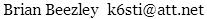# Weighted S/N

Most FM tuner manufacturers specify S/N using an unweighted measurement, but a few use A weighting. I wondered how it might affect the S/N figure. I wrote a short computer program to find out.

```FOR f = 20 TO 15000					' Integrate noise power from 20 Hz to 15 kHz
d = 1 / (1 + (2 * 3.141593 * 75E-6 * f) ^ 2)		' Deemphasis filter power factor
m = d * f ^ 2						' Mono noise power
s = m + d * ((38000 - f) ^ 2 + (38000 + f) ^ 2)	' Stereo noise power
um = um + m						' Unweighted mono sum
us = us + s						' Unweighted stereo sum
a = f ^ 2						' w = A-weight power factor
w = (13681.783 * a) ^ 4 / ((a + 424.36) * (a + 148693636)) ^ 2 / (a + 11599.29) / (a + 544496.41)
wm = wm + w * m					' Weighted mono sum
ws = ws + w * s					' Weighted stereo sum
NEXT f
PRINT 10 * LOG(wm / um) / LOG(10)			' Result in dB
PRINT 10 * LOG(ws / us) / LOG(10)			' LOG is natural log
```

The result is

```Mono:   A-weighted S/N = unweighted S/N + 1.3 dB
Stereo: A-weighted S/N = unweighted S/N + 0.4 dB
```

The calculations assume that the test tone frequency is 1 kHz, the deemphasis time constant is 75 microseconds, the composite response is flat, and composite noise rises 6 dB per octave. The result is insensitive to composite droop.

October 6, 202088–108 MHz